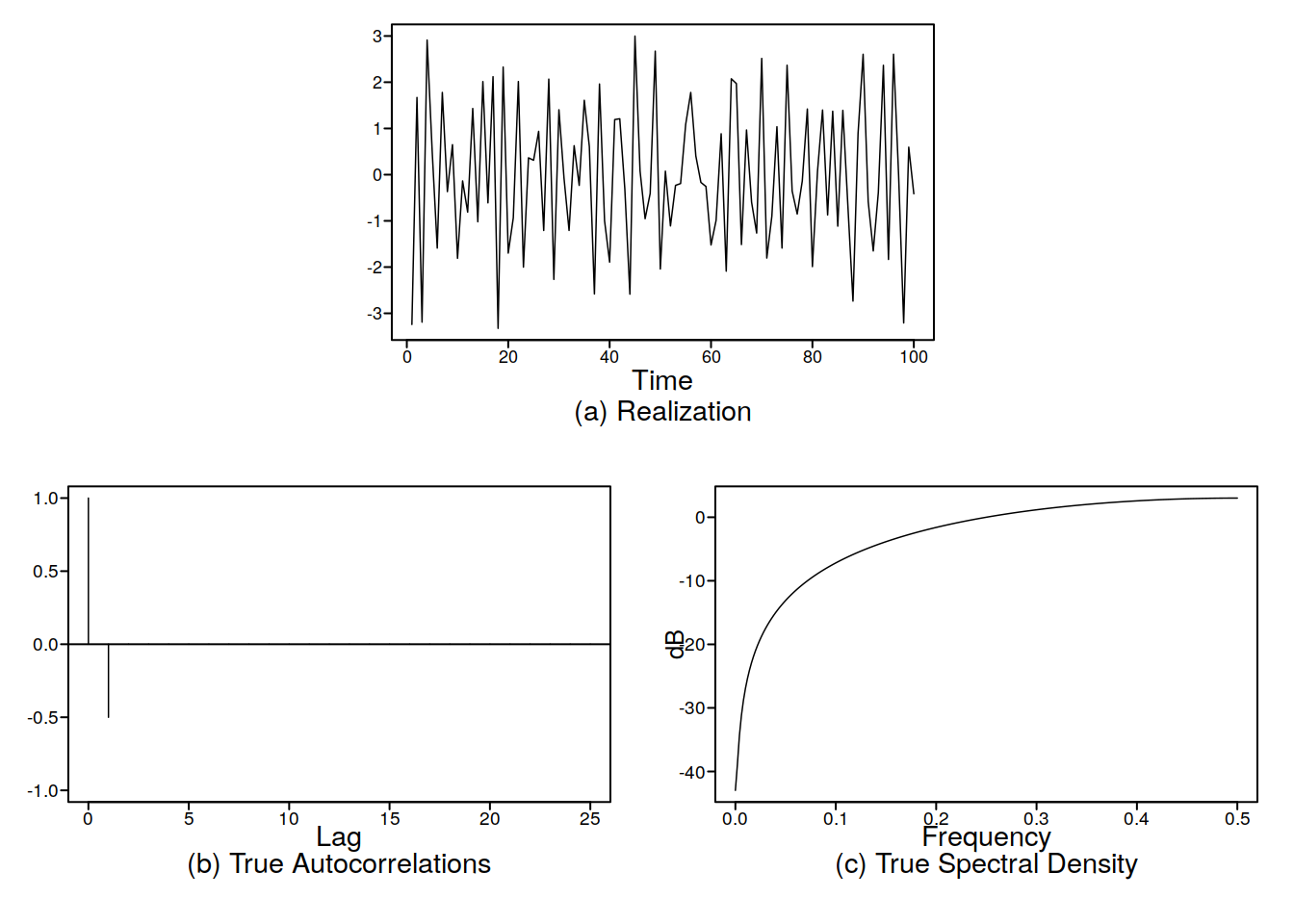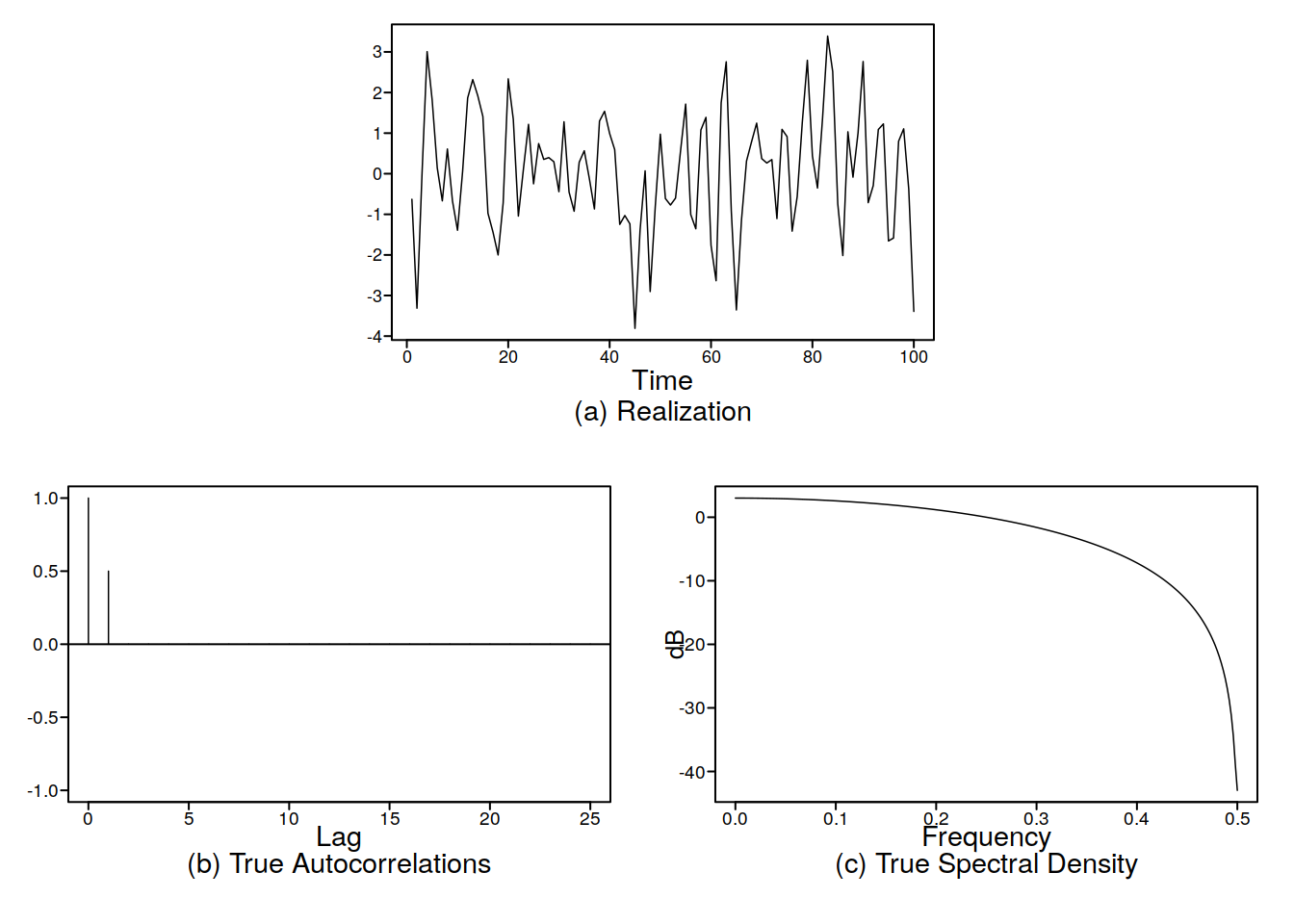# Unit 15 Some examples of MA(1)

### 15.0.1 Positive theta1

xs <- gen.arma.wge(n = 200, theta = 0.99)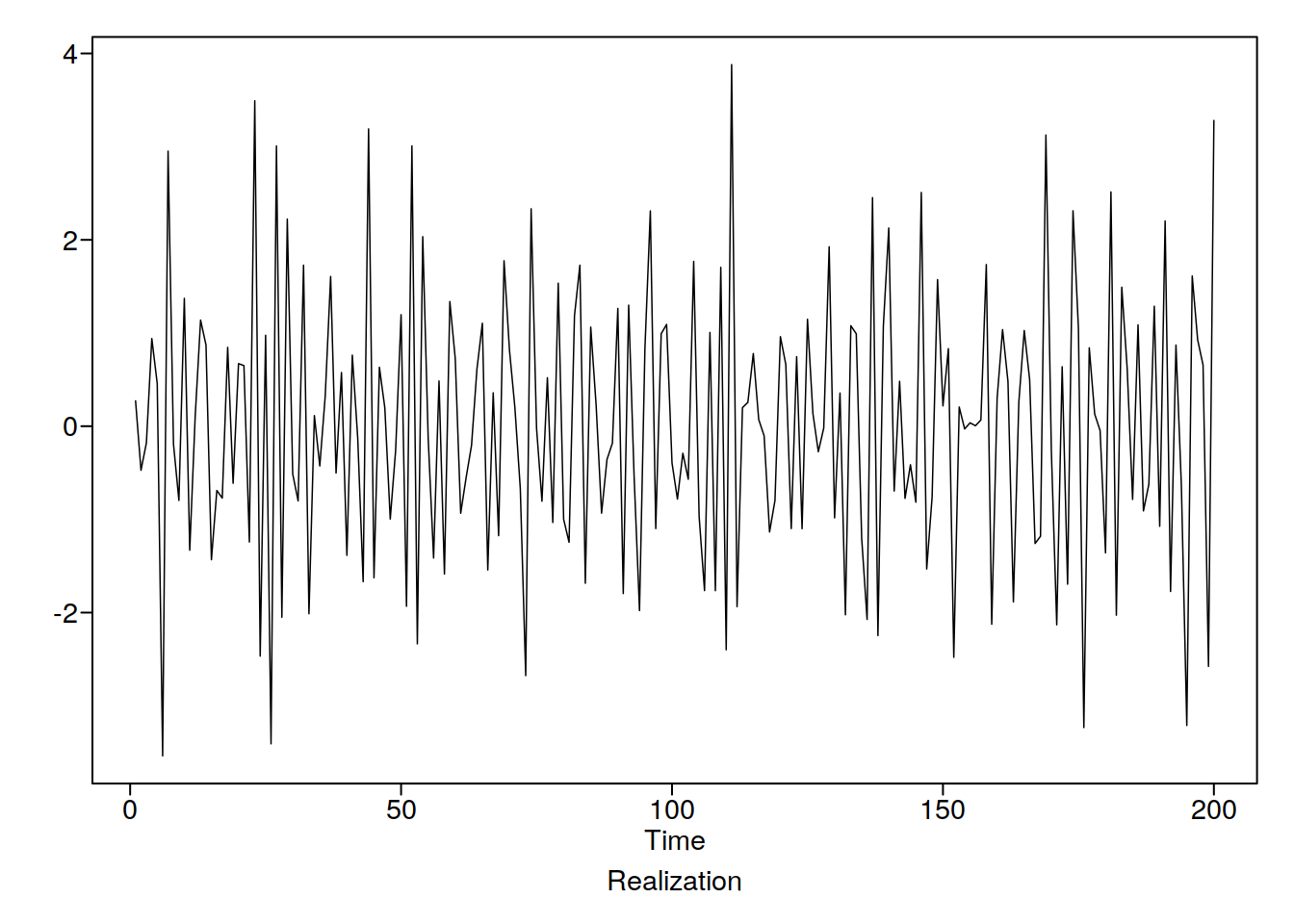Lets check this out now

ggacf(xs) + th
parzen.wge(xs)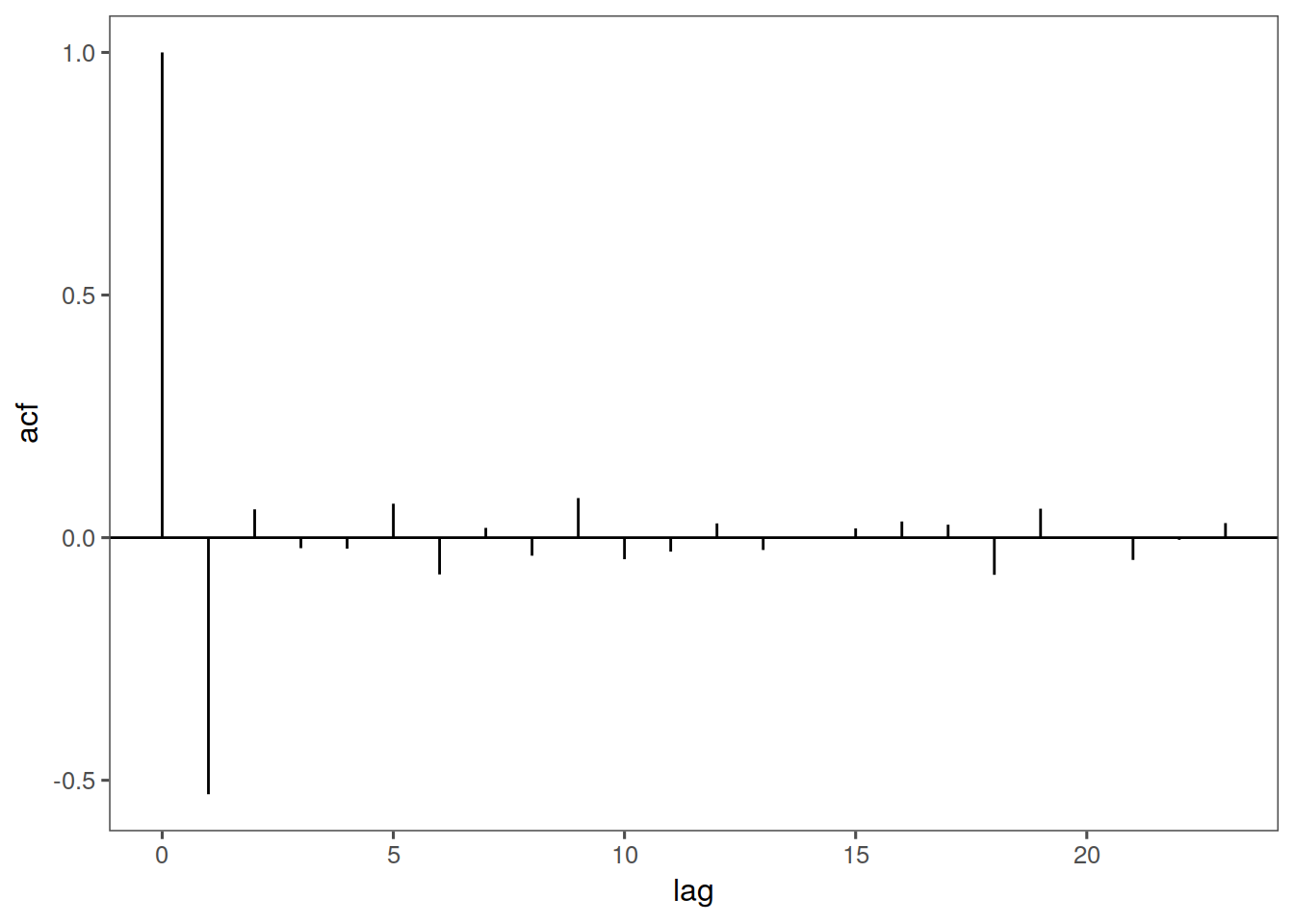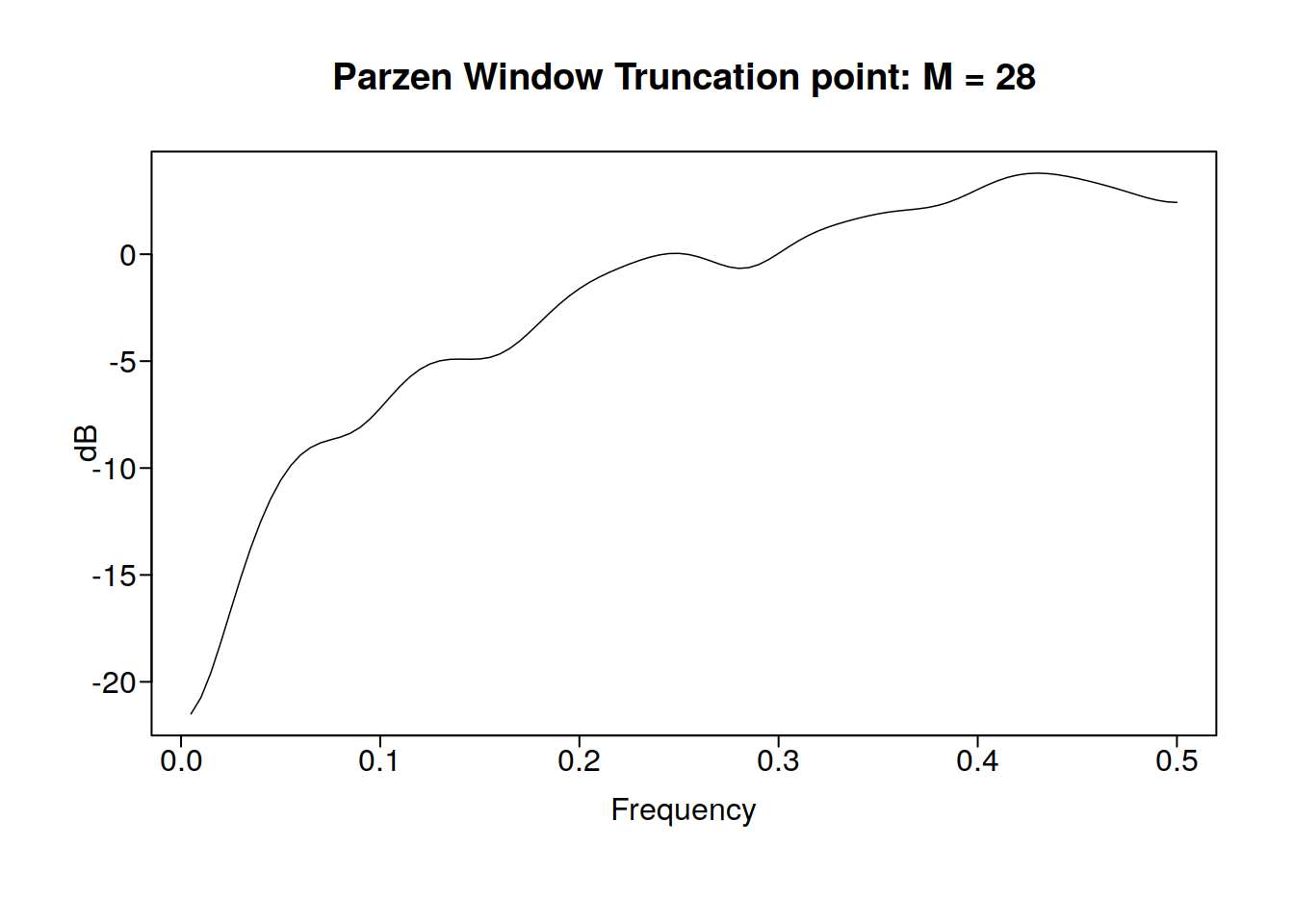Lets get some math done too and check out the ACF at lag 1

macf1 <- function(theta) {
num <- -theta
denom <- 1 + theta^2
num/denom
}
macf1(0.99)
#  -0.4999747

From this we can also see that the maximum possiblevalue of autocorrelation at lag 1 is 0.5.

### 15.0.2 Negative theta

Now lets try it out with a negative theta:

xs <- gen.arma.wge(n = 200, theta = -0.99)
ggacf(xs) + th
parzen.wge(xs)
macf1(-0.99)
#  0.4999747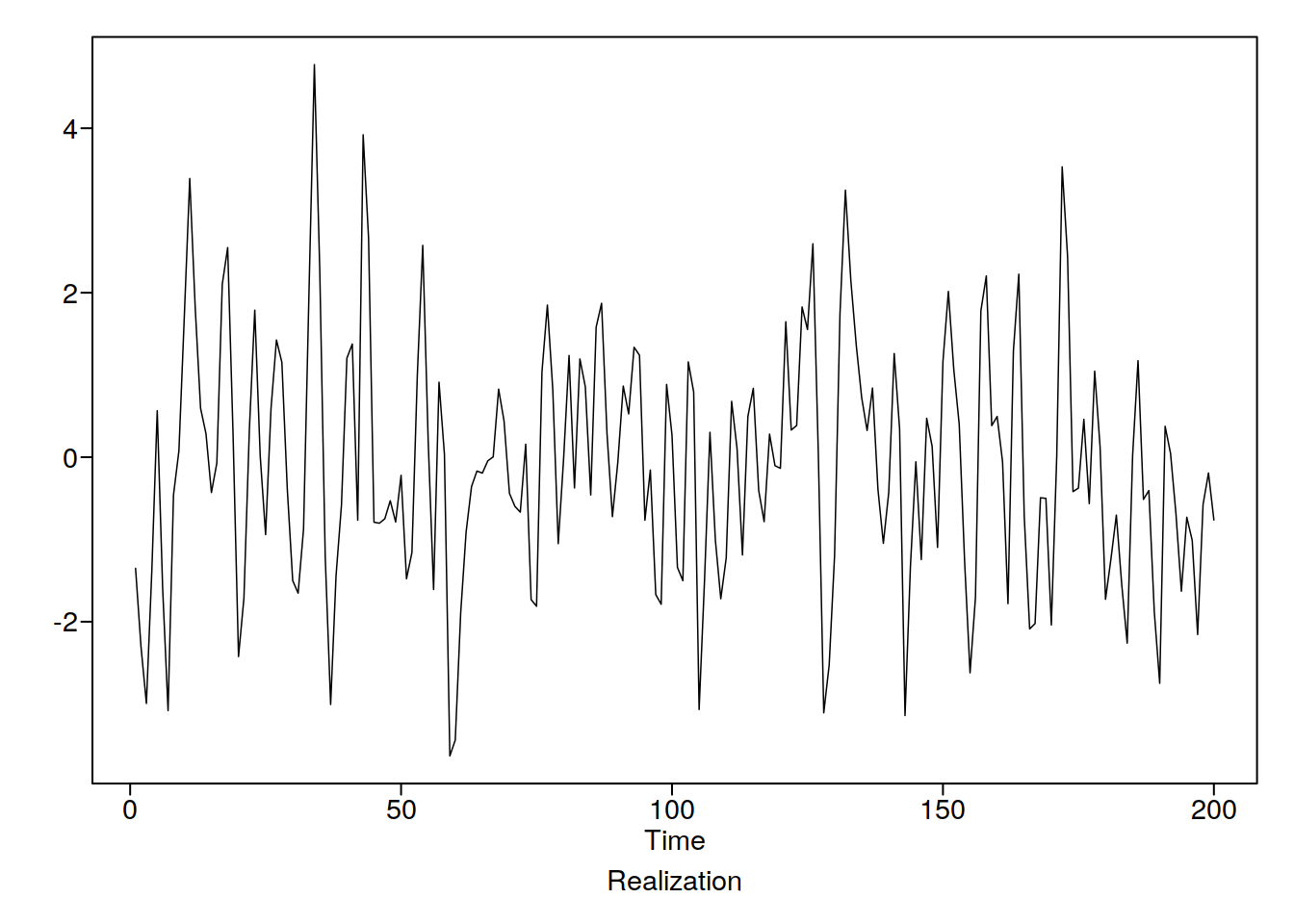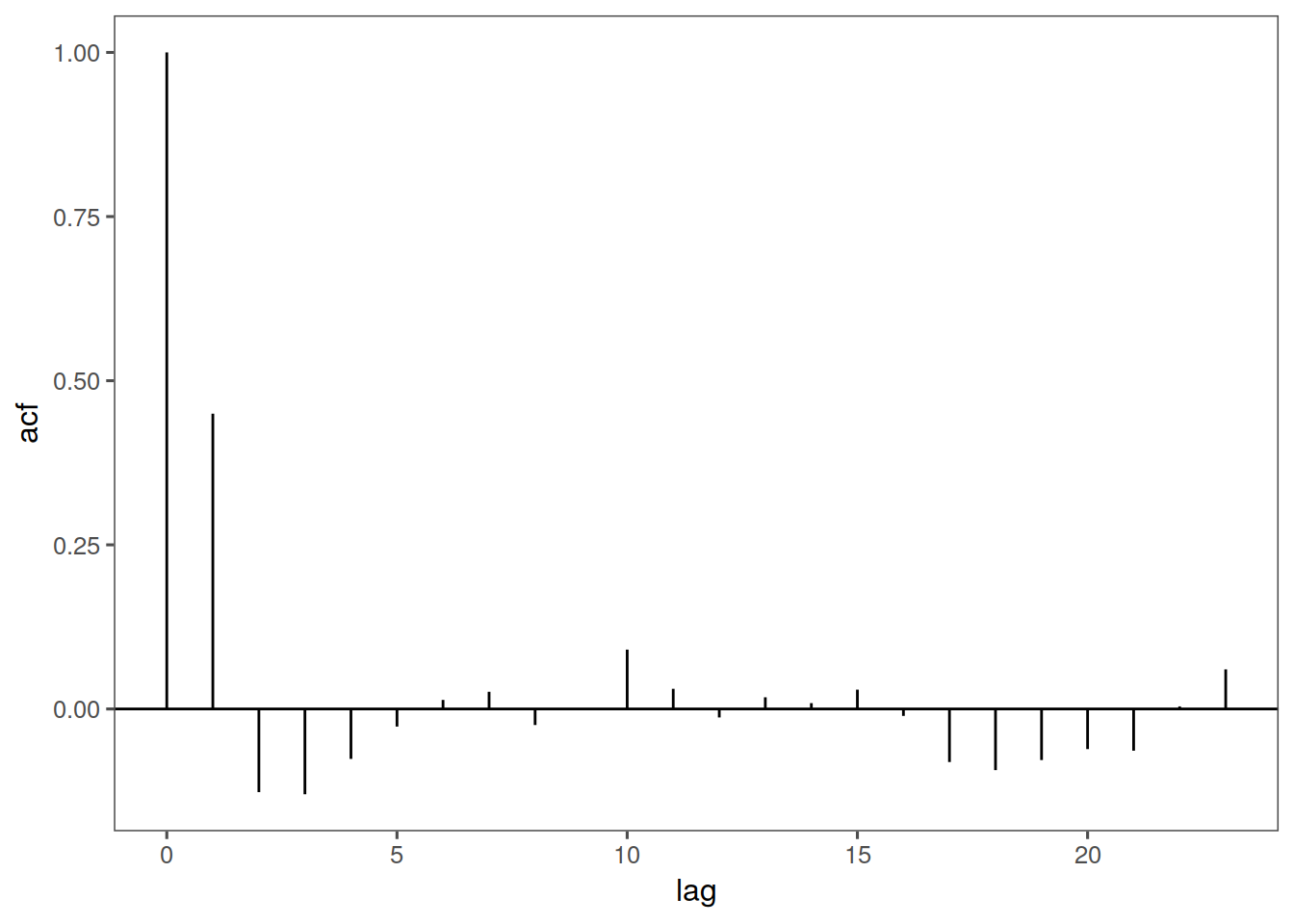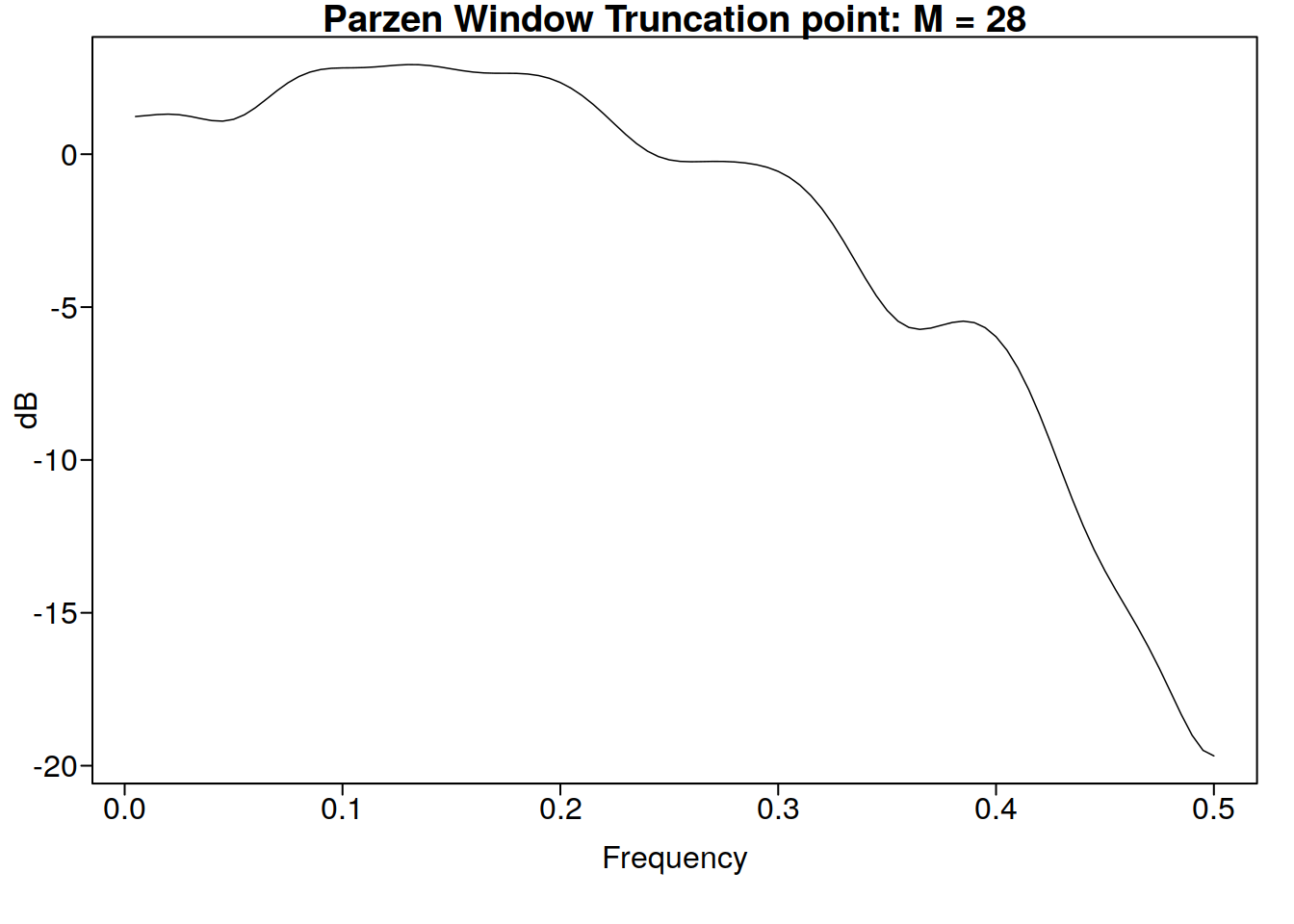Note: the negative autocorrelation of a positive theta, as well as the dip at 0 in the spectral density tell us it is going to oscillate a bit more than the negative theta. Lets check out the true autocorrelations and spectral densities of these models:

plotts.true.wge(theta = 0.99)
plotts.true.wge(theta = -0.99)